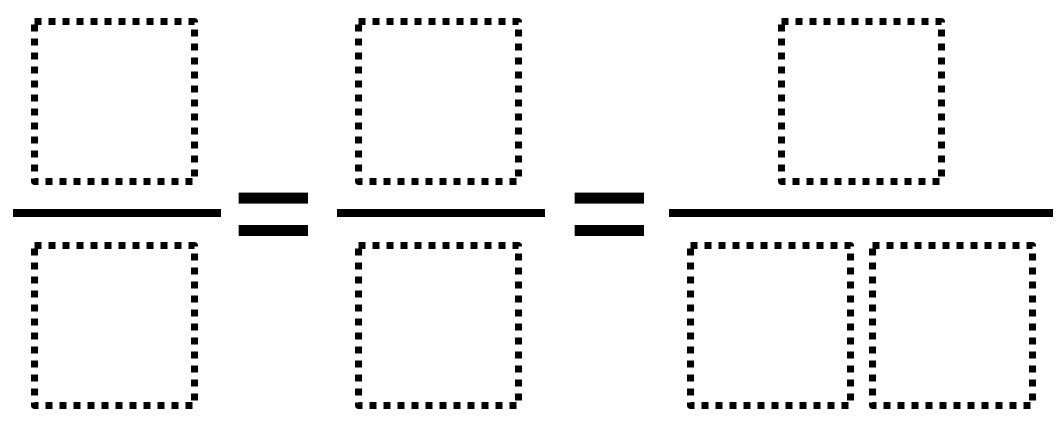Home > Grade 4 > Equivalent Fractions

# Equivalent Fractions

Directions: Use the digits 1 to 9, at most one time each, to make three equivalent fractions.### Hint

What must be true about all three fractions?

There are three possibilities:
6/8 = 3/4 = 9/12
2/4 = 3/6 = 9/18
2/8 = 1/4 = 9/36

Source: Owen Kaplinsky

## Multiplying Two-Digit Numbers – Closest to 7,000

Directions: Directions: Using the digits 1 to 9 at most one time each, fill in …

1.As far as my class could figure out the answers you present are the only three possibilities. We worked on creating organized lists to rule out all other possibilities.

-no numerators or denominators greater than 4 will work because when you multiply them by 2 they become a two digit number and we need two fractions with single digit denominators (i.e. 2/5 x 2= 4/10 two digit numerator – 2/6, x 2 = 4/12 – etc)
-The only possibilities for most simplified fraction to begin with are 1/2, 1/3, 1/4, 2/3, 3/4 – and 1/3 and 2/3 do not work
– 1/2: use 2/4, 3/6, 9/18
-1/3 doesn’t work
-1/4: 1/4, 2/8, 9/36
-2/3 – doesn’t work
-3/4: 3/4, 6/8, 9/12

•Thanks. Just updated it.

•2/5 x 2 = 4/5 not 4/10.
2/6 x 2 = 4/6 not 4/12

2.I am curious why this task is tagged with Common Core 3.NF since Grade 3’s expectation in NF are limited to fractions with denominators of 2, 3, 4, 6, and 8. Would this task be better suited for Grade 4 where students work with denominators of 2, 3, 4, 5, 6, 8, 10, and 12?

•Jennifer Houghton

Agree….I’ll use it for 4th grade. It’s a great one for that grade level.

•Good point. I changed it.

3.That could work, but I think it is fine. Thanks for your opinion!

4.1/2=3/6=4/8

•J/k, I overlooked the second blank 🙂

5.im not understanding how we are supposed to show are kids how to do this work with out any examples or any understanding on how to do this

6.2/6=3/1=4/12

•Liudmila homolka

3/1 is not = 2/6 and 4/12

7.500000/1000000=1/2=300/600

8.9.10.I have the name Owen too!

11.The 10 has a zero which isn’t in the 1 to 9 realm

12.2/4=3/6=9/18

13.If you allow the option of the digit zero, there are two more possibilities:
2/4 = 3/6 = 5/10
4/8 = 3/6 = 5/10

In fact, why not allow the possibility of zero? It’s important that students begin thinking about it? Is that too much for a 4th grader to deal with in fractions? (I have no idea–I teach high school.)